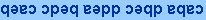CH223 - 11:00                            EXAM-1B                                Practice

 Information: gas constant:  R = 0.08206 L·atm/mole·K = 8.314 J/mole·K Avogadro's number:  NA = 6.022 ´ 1023/mol van't Hoff equation:  ln(K2/K1)= -(DH/R)(1/T2 -1/T1) normal freezing point of water:   0.00°C = 273.15K water ionization constant (25°C):  Kw = 1.00 ´ 10-14 pressure:  1 atm = 760 mmHg = 760 torr exp(x) = ex,  ln(ex) = x,  log(10x) = x quadratic equation:   ax2 + bx + c = 0,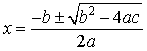Multiple choice:  select one answer for each question:

1.  Calculate Kc for A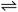2B + C if [A] = 0.125 M, [B] = 0.862 M and [C] = 0.642 M at equilibrium.

 a) 2.86 b) 4.43 c) 3.82 d) 5.36

2.  An aqueous solution is basic if the condition ______ is satisfied.

 a) [H3O+] < [OH-] b) [H3O+] = [OH-] c) [H3O+] > [OH-]

3.  For which of the following values of Kc does the reaction go the most toward completion?

 a) 1012 b) 1025 c) 10-25 d) 10-12

4.  The relationship between Kc and Kp for the reaction A(s)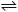B(g) + C(g) at 25°C is
 a) Kc = 1.67 ´ 10-3 Kp b) Kc = 24.5 Kp c) Kc = 4.09 ´ 10-2 Kp d) Kc = 1.63 ´ 10-7 Kp e) Kc = 598 Kp

5.  The pH of 5.26 × 10-6 M HCl is

 a) 6.41 b) 5.15 c) 3.63 d) 5.28 e) 4.94

6.  Kp = 0.17 for A(g)2B(g).  Which way does the reaction go when PA = 0.30 atm and PB = 0.25 atm?

 a) A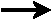2B b) A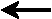2B c) no change, system is at equilibrium

7.   If Kc = 0.962 for A + 2B3C, then  Kc for 6C4B + 2A would equal

 a) 1.03 b) 0.98 c) 1.04 d) 0.93 e) 1.08

8.  HClO has Ka = 3 ´ 10-8.  Determine Kb for ClO-.

 a) 3 ´ 10-6 b) 3 ´ 10-22 c) 3 ´ 10-7 d) 3 ´ 10-9 e) 3 ´ 10-15

9.  Calculate [B] in an equilibrium mixture that has [A] = 0.500 M,  [C] = 0.125 M:

A(g)B(g) + C(g) ,     Kc = 4.38 ´ 10-5

 a) 5.6 ´ 10-5 M b) 2.7 ´ 10-6 M c) 7.0 ´ 10-4 M d) 1.8 ´ 10-4 M e) 1.1 ´ 10-5 M

10.  The reaction  A(g)B(g) + C(g)  is endothermic.  The highest yield of products is obtained at

 a) low temperature, high pressure b) high temperature, high pressure c) low temperature, low pressure d) high temperature, low pressure

11.  At equilibrium the forward (1) and reverse (-1) rates of the reaction 2AB satisfy

 a) Rate1 = 2 Rate-1 b) 2 Rate1 = Rate-1 c) Rate1 = 4 Rate-1 d) 4 Rate1 = Rate-1 e) Rate1 = Rate-1

12.   The pH of a 2.48 × 10-3 M solution of the soluble salt Ba(OH)2 is

 a) 11.7 b) 9.30 c) 10.6 d) 9.52 e) 8.48

13.   The concentration of F- in 0.65 M HF (Ka = 6.80 ´ 10-4) is:

 a) 3.2 ´ 10-2 M b) 2.1 ´ 10-2 M c) 6.8 ´ 10-3 M d) 2.4 ´ 10-2 M e) 0.65 M

14.  Which statements are TRUE?

1 = O2
- is the conjugate base of the hydroxide ion.
2 = Water is amphoteric.
3 = H3PO4 is the conjugate acid of H2PO4-

 a) 1 only b) 2 only c) 3 only d) 1 and 3 only e) 1, 2, and 3

15.  The concentration of hydronium ion in 0.125 M NH3 (Kb = 1.8 × 10-5) is

 a) 5.42 ´ 10-12 M b) 6.13 ´ 10-12 M c) 4.80 ´ 10-11 M d) 6.67 ´ 10-12 M e) 7.25 ´ 10-12 M

16.  The correct order of increasing base strength in water is expected to be

 a) F- < Cl- < Br- b) Cl- < F- < Br- c) Br- < Cl- < F- d) F- < Br- < Cl- e) Br- < F- < Cl-

17.  NH3 in the reaction Ag+ + 2 NH3Ag(NH3)2+ is a  ______
1 = Arrhenius base
2 = Bronsted-Lowry base
3 = Lewis base

 a) 1 only b) 2 only c) 3 only d) 2 and 3 only e) 1, 2 and 3

18.   Sulfurous acid H2SO3 is a weak diprotic oxoacid.  The concentrations of species in a 0.25 M solution
of H2SO3 are expected to satisfy

 a) [H2SO3] > [HSO3- ] > [SO32- ] b) [H2SO3] < [HSO3- ] > [SO32- ] c) [H2SO3] > [HSO3- ] < [SO32- ] d) [H2SO3] < [HSO3- ] < [SO32- ] e) [H2SO3] = [HSO3- ] > [SO32- ]

19.  Use the acid constants for H2S (pKa1 = 7, pKa2 = 17) and HIO (pKa = 11)  to determine  Kc for
IO-(aq) + HS-(aq)HIO(aq) + S2-(aq)

 a) 1 ´ 10-28 b) 1 ´ 10-9 c) 1 ´ 10-14 d) 1 ´ 10-8 e) 1 ´ 10-6

20.  Calculate the degree of ionization of HIO3 in 0.16 M HIO3 (Ka = 0.24).  HINT:  use quadratic equation.

 a) 78% b) 69% c) 58% d) 64% e) 73%

 KEY: